• ### trigger object not working as expected

The left part of patch is working as expected while the right hand part is not. why the object trigger is not turning the toggle on and off?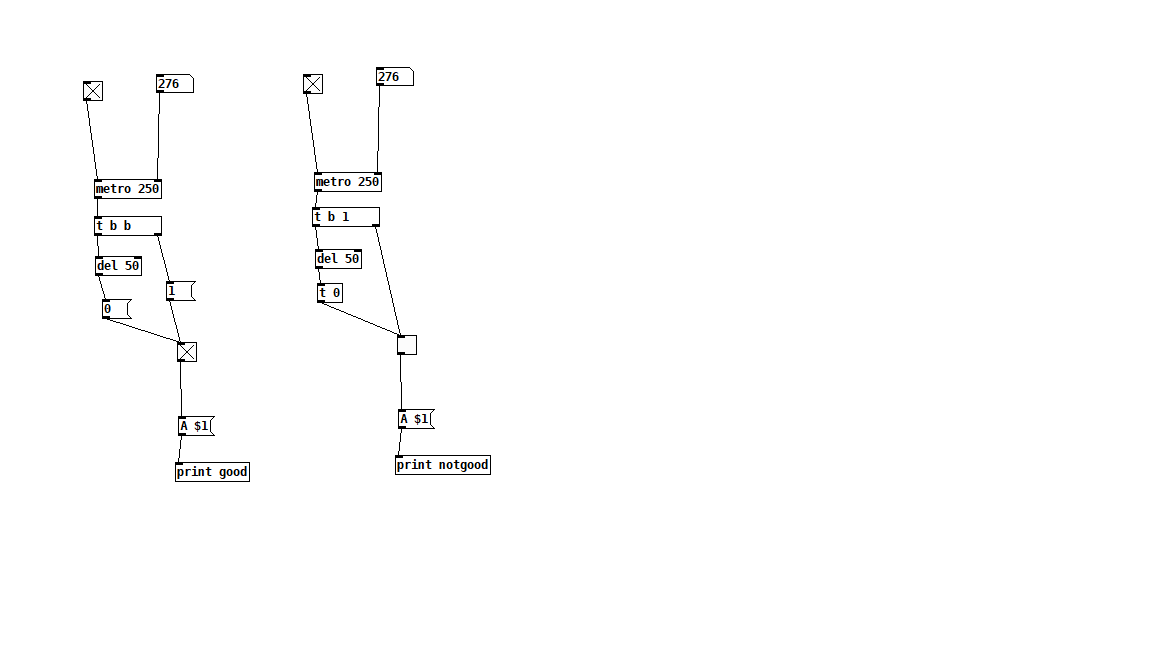• Posts 10 | Views 271
• and the second part of the question:

I try to send the message A 0 or A 1 to the arduino via serial object but I'm getting an error. What am I missing?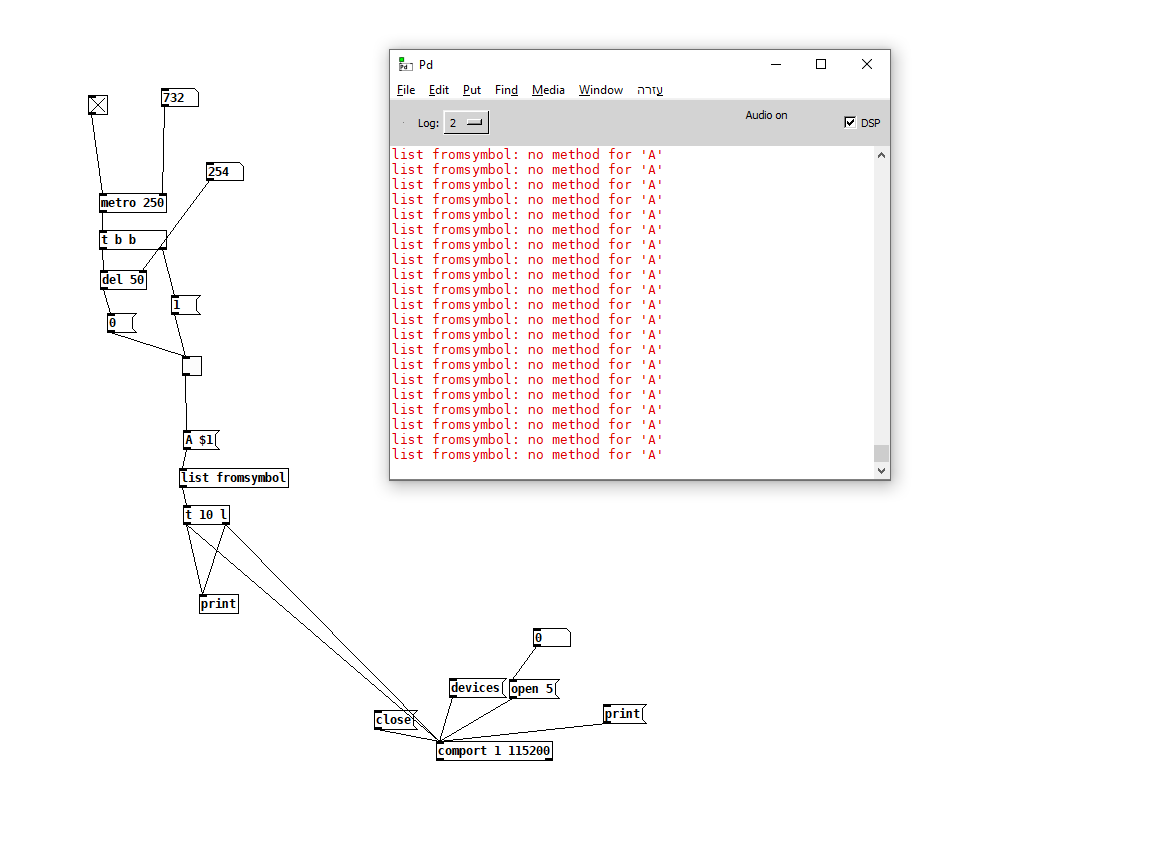and my arduino code:

``````const int LED_pins[] = {11, 12, 13};
const int NUM_LEDS = 3;

void setup() {
for (int i = 0; i < NUM_LEDS; i++) {
pinMode(LED_pins[i], OUTPUT);
}
Serial.begin(115200);
}

void loop() {
if (Serial.available() > 0) {
int state = Serial.parseInt();
if (id == 'A') {
digitalWrite(LED_pins, state);
} else if (id == 'B') {
digitalWrite(LED_pins, state);
} else if (id == 'C') {
digitalWrite(LED_pins, state);
}
}
}
``````
• @KMETE For the [toggle] I am pretty sure [t b 1] will send a 0 instead of a 1. In fact any float value will send 0.
The correct parameters for [toggle] are f b s l p a (float, bang, symbol, list, pointer, anything).

[[list fromsymbol] expects a symbol obviously, but what is not obvious is that symbols need to be tagged explicitly as a symbol or they are simply "text"...... it drives us nuts sometimes.
So you need the message [symbol A \$1(
But that will not work...... as it will only receive the A because of the space afterward...... so you will have to stick the \$1 to the A....... [symbol A\$1( will produce 65 48 (for 0) and 65 49 (for 1).
David.

• This is what I'm getting when do the following: (not quite what I am expecting)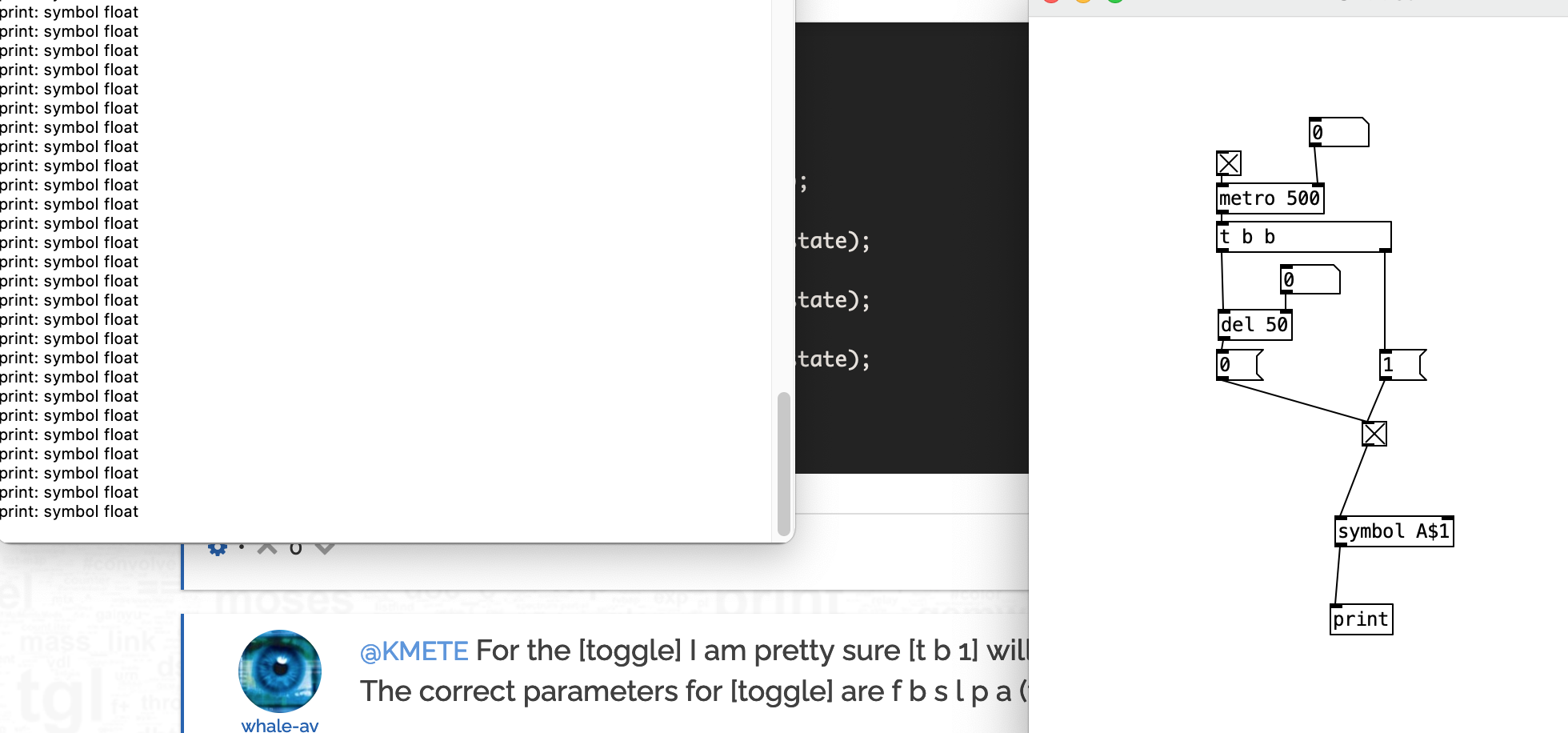• and that is what I'm getting when added [list fromsymbol] (as well not quite what I would expect it)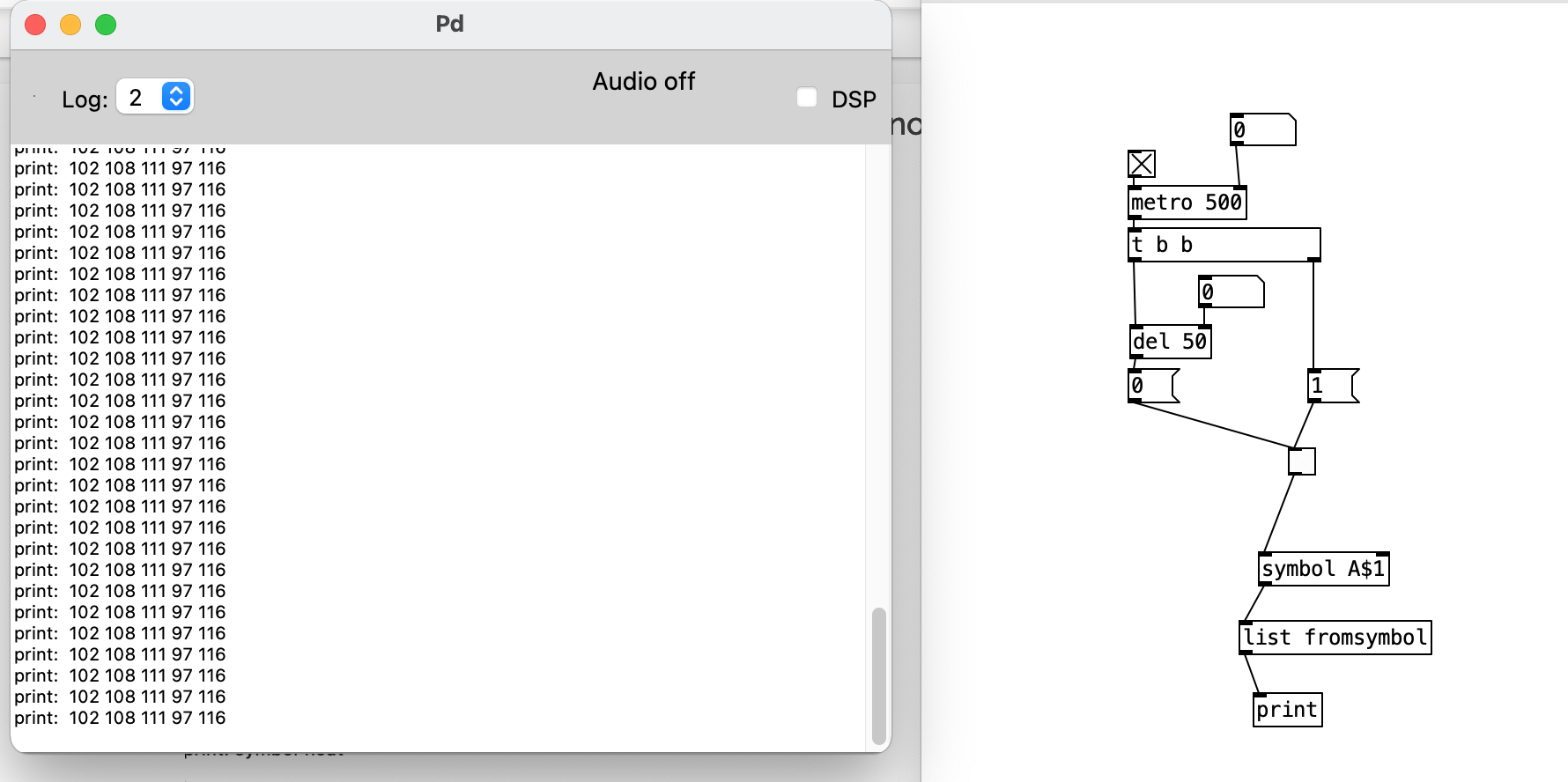• @KMETE It needs to be a message...... [symbol A\$1(...... not an object [symbol A\$1]
David.

• Thanks working like a charm! for some reason the output in arduino was flipped so I just change this:

``````if (id == 'A') {
digitalWrite(LED_pins, state);}
``````

to this:

``````if (id == 'A') {
digitalWrite(LED_pins, !state);
``````
• @KMETE I don't "know" but it could be that the leds are turned on by "pull down".
For that the leds are connected between the +Ve rail and the pin and turn on when the Pin goes to 0V.
When the pin is high (+ve) the led is off.
That might explain the reversal.
Maybe?
The pin can be at 0V or 3.3V (logic volts). Using pull down you can get 5V (between 0V and the 5V power rail). Useful for relays (if there are no opto-isolators on the relay board), but for a led you only need 0.7V and a current limiting resistor so not necessary..... but.... I don't know how they are wired for the Arduino.
David.

• how can I send data back from arduino to pd while transfering data from pd to arduino?

so when I'm sending A 1 to arduino I'm turning led ON and I would like to receive in pd the message "Led1 On" in pd

• solved it:

``````const int LED_pin[] = {13, 12, 11};
const int NUM_LEDS = 1;
const char LED_ids[] = {'A', 'B', 'C'};

void setup() {
for (int i = 0; i < NUM_LEDS; i++) {
pinMode(LED_pin[i], OUTPUT);
}
Serial.begin(115200);
}

void loop() {
if (Serial.available() > 0) {
int state = Serial.parseInt();
for (int i = 0; i < NUM_LEDS; i++) {
if (id == LED_ids[i]) {
digitalWrite(LED_pin[i], !state);
Serial.print("led");
Serial.println(i + 1);
Serial.print("");
Serial.println(!state);
break;
}
}
}
}

``````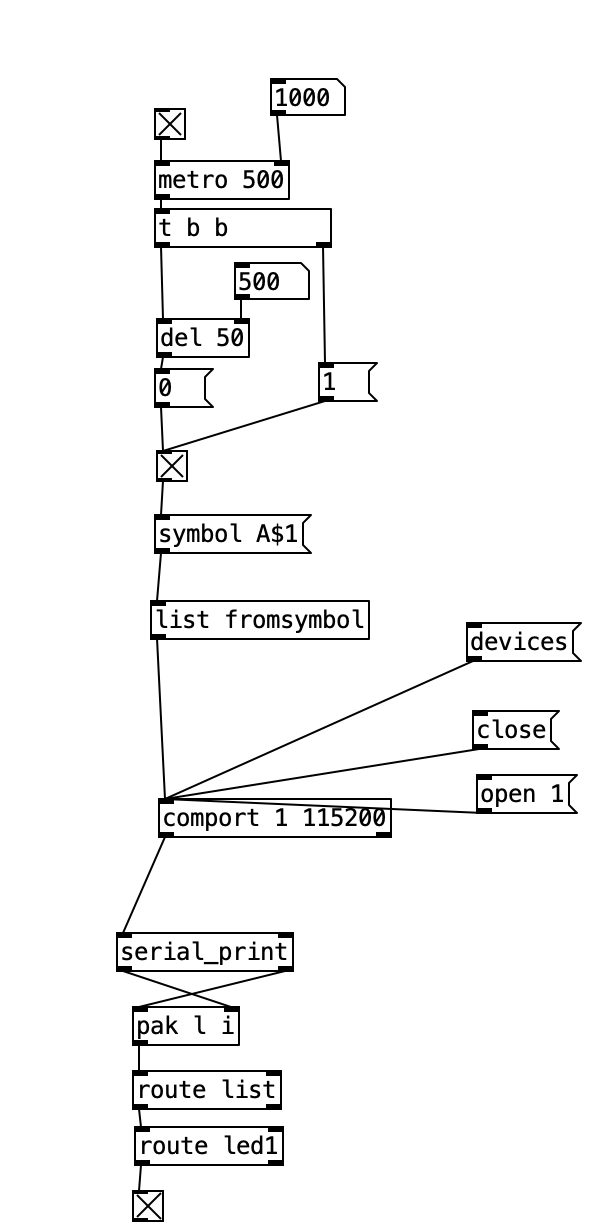Posts 10 | Views 271
Internal error.

Oops! Looks like something went wrong!